Burlay O. I., Kuznetsov K. A., Atanova M. Y.

Oles Honchar Dnipropetrovsk National University

INTELLIGENT SYSTEM FOR SUPPORTING DECISION-MAKING FOR DISPATCHING LIGHT OIL

Problem statement

"Dnepronefteprodukt" delivers light oil at gas stations by gasoline tankers. We need to make a decision about the importation of light oil products at some gas stations. We also need to find the shortest route f or oil tankers.

The designations

N – the total number of stations

F – the total number of fuel types

fq – the total cost of q-th fuel type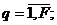ciq , diq – the volume and "dead" remains of the q-th tank on the i-th station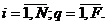t0 – the current time

riq (t) –the remaining fuel in the q-th tank at the i-th station at time t.

wiq (t0±p) – the cumulative volume of the imported q-th fuel at the i-th station at time t0±p;

siq (t0±p) – the daily sales of the q-th fuel at the i-th station at time t0 ± p. t0±p.

There is a relationship: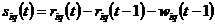, (1)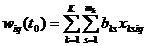φiq – the time until the selling of fuel from the q-th tank from the i-th station stops.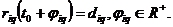(2)

– the total number of fuel trucks;

– the cost of 1 km run of any fuel truck;

mk – the number of sections of the k-th fuel truck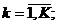bsk – the volume of s-th section of the k-th fuel truck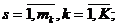ρij – the distance in kilometers from the i-th to the j-th station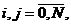(0 – the index of the tank farm)

We should find the two sets of Boolean variables xksiq and ykij.

The mathematical model

Let us formulate the following restrictions: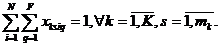(3)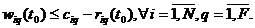(4)

The variables are related by the following relation: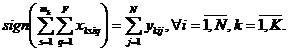(5)

We also need to define a route that begins and ends at the oil tank.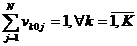(6)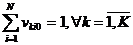(7)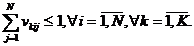(8)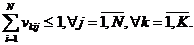(9)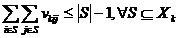(10)

Where Xk – the set of the indexes of the stations, that are visited by k-th fuel truck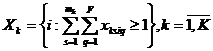. (11)

Optimality criterion

From a mathematical point of view, this problem is the task of the two- criterion optimization:

The first criterion is the integral criterion: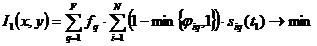. (13)

And the second criterion: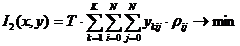. (14)

Solution to the problem

We created the graph of the routes to find the distances matrix (ρij). It was constructed on the basis of GIS OpenStreetMap ©. We also used the Floyd–Warshall algorithm for searching the shortest path. (APSP, All-pairs shortest paths). The complexity of the algorithm is Θ(n3), so we had to use nvidia graphics accelerator.

This is the statistical model: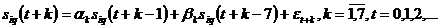(15)

It consists of seven separated ARIMA-models (according to the 7 days of the week).

It demonstrates no more than 5% deviation from the observed data for more than 97% of the time series, and therefore it is acceptable for practical use.

The next model shows the dynamics of remaining fuel: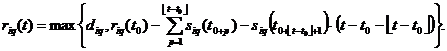(16)

The examples given above allow us to calculate the criterion (14) value with all constraints (3)-(11) and (13) for any sets.

The dimension of the search space of our problem grows exponentially with the number of the stations. The use of random search leads to different solutions for the same input data. This is explained by the fact that each of these approaches has a probabilistic component.

Conclusion

The proposed optimization criteria are robust to changes in the input data. The successful experience of using the system shows that the current system may be applicable to a wide range of problems in transport logistics in Ukraine.